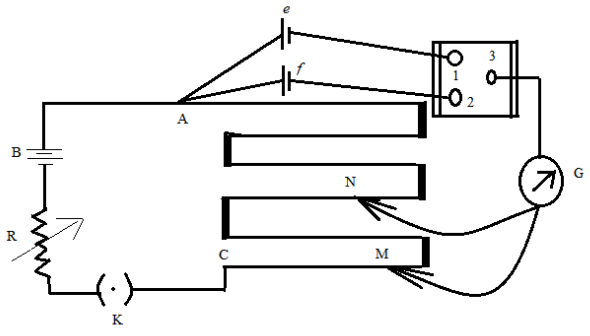QuestionAnswers

# Draw a labelled circuit diagram of a potentiometer to compare emfs of two cells. Write the working formula (Derivation not required).Hint: A potentiometer consists of a galvanometer, a variable resistance, a battery. In the circuit for the comparison of the emfs of the two cells, the circuit will comprise two cells as well.

Complete step by step solution:Figure: A potentiometer circuit designed for comparing emfs of two cells.
In the above figure, the wires run from point A to point C. The small vertical portions are metal strips connecting various sections of the wire. B is the battery, R is the variable resistance (the arrow indicates variable resistance) which is also known as the rheostat. e and f are the two cells whose emfs are to be compared. G is the galvanometer and N and M are the two points to which the galvanometer jockey is connected. The points marked 1,2 and 3 form a way key. K is the key of the circuit.
Working formula:
If ${{\varepsilon }_{1}}$ is the emf of cell e and ${{\varepsilon }_{2}}$is the emf of cell f, then the formula for comparison is given as,
$\dfrac{{{\varepsilon }_{1}}}{{{\varepsilon }_{2}}}=\dfrac{{{l}_{1}}}{{{l}_{2}}}$
where, ${{l}_{1}}$ and ${{l}_{2}}$ are the distances of point N and M respectively from point A.
There is also an arrangement of potentiometers for determining the internal resistance of a cell, where we do not need to incorporate the two cells.

Note: Students must note that ${{l}_{1}}$ and ${{l}_{2}}$ are the distances from point A and not from the galvanometer G or point C. And to represent the variable resistance, it is always necessary to give the arrow across the resistance, else it does not represent a variable resistance.

View Notes
Labelled Diagram of Human EarIntegrated CircuitArea of a Sector of a Circle FormulaAC Voltage Applied to Series LCR CircuitArea of a Rhombus FormulaPerimeter of a Trapezoid FormulaSummary of a Photograph - PoemCentroid of a Trapezoid FormulaReconstitution of a Partnership FirmElectric Field of a SphereImportant Questions for CBSE Class 11 Physics Chapter 4 - Motion in a PlaneImportant Questions for CBSE Class 11 Physics Chapter 3 - Motion in a Straight LineImportant Questions for CBSE Class 6 English A Pact with The Sun Chapter 1 - A Tale of Two BirdsImportant Questions for CBSE Class 6 English Honeysuckle Chapter 8 - A Game of ChanceImportant Questions for CBSE Class 11 Business Studies Chapter 7 - Formation of a CompanyImportant Questions for CBSE Class 12 PhysicsCBSE Class 7 Science Water: A Precious Resource WorksheetsImportant Questions for CBSE Class 6 English A Pact with The Sun Chapter 8 - A Pact with the SunImportant Questions for CBSE Class 6 English A Pact with The Sun Chapter 10 - A Strange Wrestling MatchImportant Questions for CBSE Class 7 English Honeycomb Chapter 2 - A Gift Of ChappalsCBSE Class 10 Hindi A Question Paper 2020Hindi A Class 10 CBSE Question Paper 2009Hindi A Class 10 CBSE Question Paper 2015Hindi A Class 10 CBSE Question Paper 2016Hindi A Class 10 CBSE Question Paper 2012Hindi A Class 10 CBSE Question Paper 2010Hindi A Class 10 CBSE Question Paper 2007Hindi A Class 10 CBSE Question Paper 2013Hindi A Class 10 CBSE Question Paper 2008Hindi A Class 10 CBSE Question Paper 2014NCERT Solutions for Class 12 Accountancy Chapter 3 - Reconstitution of a Partnership Firm: Admission of a PartnerNCERT Solutions for Class 12 Accountancy Chapter 4 - Reconstitution of a Partnership Firm:Retirement/Death of a PartnerNCERT Solutions for Class 12 English Kaliedoscope Poetry Chapter 4 Kubla Khan or a Vision in a Dream a FragmentRS Aggarwal Class 12 Solutions Chapter-7 Adjoint and Inverse of a MatrixNCERT Solutions for Class 11 Physics Chapter 4 – Motion In a PlaneRS Aggarwal Class 8 Mathematics Solutions for Chapter-18 Area of a Trapezium and a PolygonRD Sharma Class 12 Maths Solutions Chapter 7 - Adjoint and Inverse of a MatrixNCERT Solutions for Class 12 Accountancy Chapter 3 - Financial Statements of a CompanyRS Aggarwal Solutions Class 8 Chapter-18 Area of a Trapezium and a Polygon (Ex 18B) Exercise 18.2NCERT Solutions for Class 11 Physics Chapter 4 Motion in a Plane In Hindi